# JavaScript Arrow Function: The Complete Guide

Arrow functions in JavaScript have a more concise syntax for writing function expressions. Arrow Functions are one of the most effective changes in ES6/ES2015 and are widely used nowadays. Arrow functions were introduced in ES6 / ES2015, and since their introduction to JavaScript, they have changed how JavaScript code looks and works forever.

## JavaScript Arrow Function

JavaScript Arrow Function has two main benefits, which are the following.

1. A shorter syntax than typical javascript functions.
2. No binding of this keyword.

Let us see the traditional function syntax.

```function funcName() {
// func body
}

funcName()```

Now, let us see the ES6 version syntax.

```const funcName = () => {
// func body
}

funcName()```

If the function body contains just a single statement, you can omit the brackets and write it all on a single line like the following code.

`const funcName = () => your code here like multiplication`

You do not even write the return statement. It automatically returns the value. Refer to the above syntax; we can write a function like this.

```// app.js

const square = (x) => x * x;
console.log(square(21));```

See the output in the console. I am using Node.js to run the above file.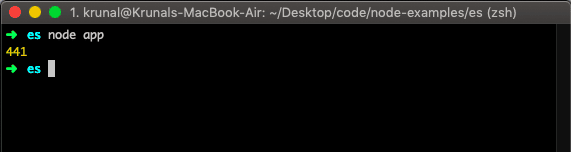Thanks to this short syntax, we can use the arrow function in small or no bodily functions, which returns in a single line of code like the above example.

If we use the traditional syntax, the code looks like this.

```// app.js

function square(x) {
return x * x;
}

console.log(square(21));```

## Breakdown of Syntax of Arrow Function

Here’s what an arrow function looks like.

`(parameters) => { statements }`

Which is equal to this in traditional syntax.

```function (parameters) {
// statements
}```

If there is no parameter, then the arrow function looks like this.

`() => { statements  }`

## How this Works In Arrow Function

A “this” keyword is a concept that can be complicated to grasp at first, and it is complicated because it changes its behavior depending on the context, like the mode of JavaScript. However, clarifying these concepts is essential because the arrow function is very different from the regular functions.

Arrow function and Traditional function works differently when defined as a method of an object. In the regular function, this refers to the object so that you can do this.

```// app.js

const Laptop = {
model: 'MacBook Air',
manufacturer: 'Apple',
fullProductName: function() {
return `\${this.manufacturer}'s \${this.model}`;
}
};

console.log(Laptop.fullProductName());
```

Output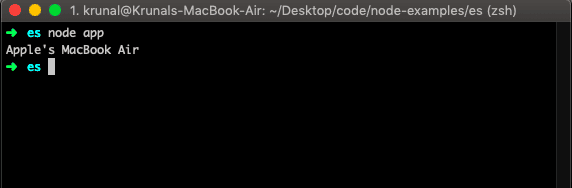Let us take the following scenario and use the arrow function inside the object.

```// app.js

const Laptop = {
model: 'MacBook Air',
manufacturer: 'Apple',
fullProductName: () => {
return `\${this.manufacturer}'s \${this.model}`;
}
};

console.log(Laptop.fullProductName());
```

Run the file and see the output.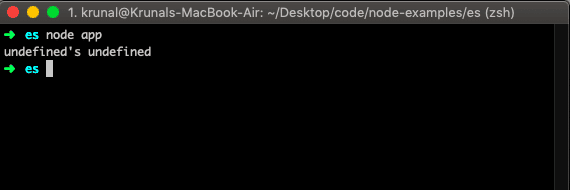That means the arrow function does not work in the Object context in ES6 because this scope is referred to as the calling function and not the function itself.

Arrow functions cannot be used as constructors when instantiating an object will raise the TypeError exception. In that scenario, the regular or traditional function is required where the dynamic context is not needed.

If you are familiar with React.js, you have faced one issue regarding this binding scope.

We faced this scope issue when we wrote the form’s textbox’s onChange() event. At that time, we need to add the function expression inside the constructor() of React.js class.

Otherwise, we do not get the textbox value inside the onChange() event’s function.

At that time, we can also use the arrow function to escape this binding keyword issue.

In the arrow function,  this is bound lexically. Simply put, this will keep its meaning from its original context.

## No binding of this

Let us see the following example.

```// app.js

function Person() {
this.age = 0;
setInterval(function() {
this.age++;
console.log(this.age);
}, 1000);
}
var user = new Person();```

In the above example, we have used Function Prototype, which behaves like a class in traditional Javascript. When we ran the example, we could not see the age in the console.

#### Output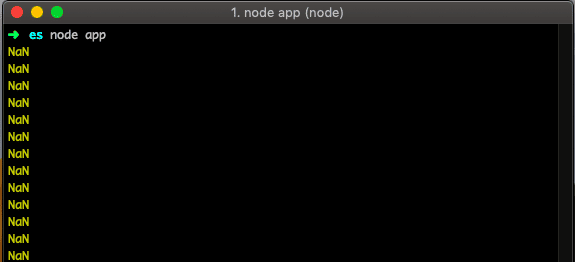That means the reference to this keyword is not lexical. To solve this problem, we can use the arrow function, and now it will begin to display the numbers.

See the following code.

```// app.js

function Person() {
this.age = 0;
setInterval(() => {
this.age++;
console.log(this.age);
}, 1000);
}
var user = new Person(); /* eslint-disable-line no-unused-vars */```

#### Output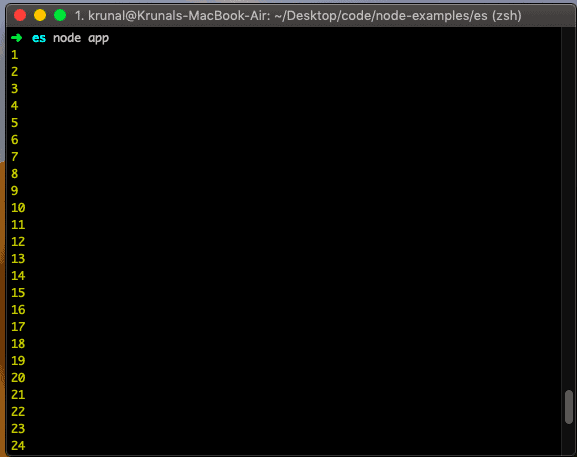An arrow function does not have its scope; it uses this value of the enclosing lexical context, i.e., Arrow functions follow the standard variable lookup rules. So while searching for this not present in the current scope, they find it from its enclosing scope. That is why we can see the output in the console.

This keyword’s behavior depends on the context; you are working on. That is why sometimes, it will not give the value you expect.

That’s it for this tutorial.

This site uses Akismet to reduce spam. Learn how your comment data is processed.